Next: Radiative Transfer Up: Physical Background Previous: Stellar Wind   Contents

# Virial Analysis

For a system to achive a mechanical equlibrium, a relation must be satisfied between energies such as potential, thermal and kinetic energies. This is called the Virial relation. For example, a harmonic oscillator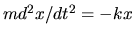has a potetial energy of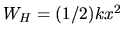and a kinetic energy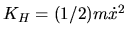. Averaging these two energies over one period, both energies give the same absolute value proportional to the oscillation amplitude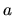squared as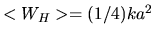and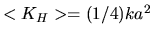. Another example is a Kepler problem. For simplicity, consider mass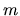running on a circular orbit with a radiusfrom a body with a mass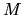. The gravitational and kinetic energies are equal to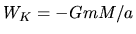and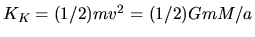, where we used the centrifugal balance as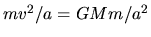. As a result, for the harmonic oscillator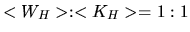while for the circular Kepler problem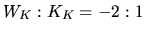. This ratio is known to be related to the power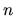of the potetial as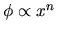. Important nature of the self-gravity is understood only with this relation without solving the hydrostatic balance equations. In the following, we describe the Virial relation satisfied with isolated systems such as stars.

Hydrodynamic equation of motion using the Lagrangean time derivative [eq.(A.3)] is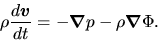(2.104)

For simplicity, consider a spherical symmetric configuration. The equation of motion is expressed as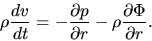(2.105)

Multiplying radius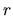to the equation and integrating by the volume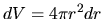over a volume from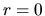to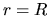, we obtain the Virial relation as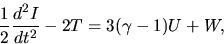(2.106)

where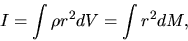(2.107)

is an inertia of this body, and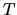and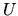are, respectively, the kinetic and thermal energies as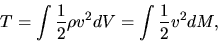(2.108)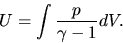(2.109)

Further,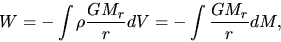(2.110)

is a gravitational energy, where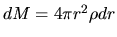and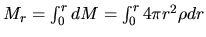Since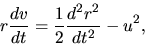(2.111)

the lefthand-side of equation (2.105) is rewritten as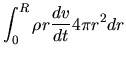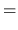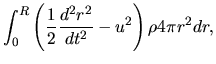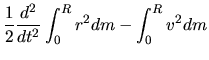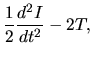(2.112)

using equations (2.107) and (2.108).

On the other hand, the first term of the rhs of equation (2.105) becomes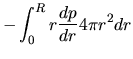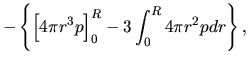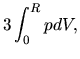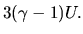(2.113)

To derive this equation, we have assumed the pressure diminishes at a radiusand the surface pressure term does not appear in the final expression. This is valid for an isolated system such as a star.

The last term of the rhs of equation (2.105) is written as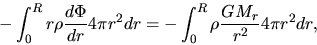(2.114)

wher we used equation (2.13). This is rewritten as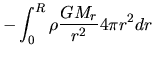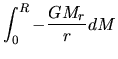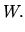(2.115)

The energy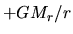per unit mass is necessary for a gas element to move from the radius, inside which mass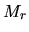is contained, to the infinity. Adding the energy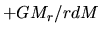for all the gas, the potential energy is obtained. In the case of a star composed of uniform density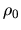,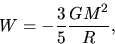(2.116)

where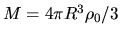.

To obtain a condition for the mechanical equilibrium, we assume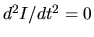. Equation (2.106) becomes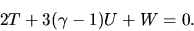(2.117)

Assuming the stystem is static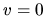, the above equation reduces to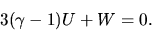(2.118)

In the case of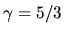this reduces to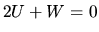. The total energy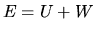is expressed as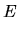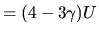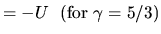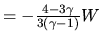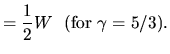(2.119)

For the gas with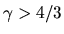, equation (2.119) gives a negative total energy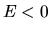and the system is in a confined state. However, if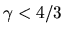, the gravity can not confine the gas.

For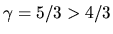, equation (2.118) gives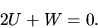(2.120)

This shows us a strange nature of the self-gravitating gas (see Fig. 2.10). That is, if the heat flux flows outward, the total energy decreases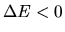. The system must contract and equation (2.119) indicates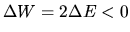(the gravitational energy decreases: the absolute value of the gravitational energy increases). However, for the thermal energy, equation (2.120) indicates that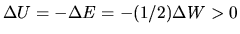for this system to be static. This shows that if the heat flux flows out from the system the thermal energy increases in the self-gravitating system. This comes from the contraction due to the gravity.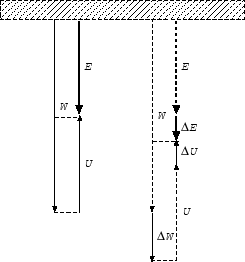Next: Radiative Transfer Up: Physical Background Previous: Stellar Wind   Contents
Kohji Tomisaka 2007-07-08